Go Back

# Learn How and When to Do a VLOOKUP in Excel

The VLOOKUP function is designed to search the value vertically in the first column of cells range or data table and returns the value from any of the columns to right. This function is, by far, one of the most useful functions when navigating a large set of data.

You need to carefully follow VLOOKUP function syntax as given below:

`=VLOOKUP (lookup_value, table_array, col_index, [range_lookup])`

lookup_value – The value to be searched for in first column of a table data range.

table_array – The data table from which to retrieve related information of a value.

col_index – The column in table_array from where to retrieve related information.

range_lookup – [optional]

TRUE = approximate match (default). It returns next smaller value if no exact match is found.

FALSE = exact match.

Following points must be remembered while using VLOOKUP function.

• Data range or table_array must be organized in vertical rows, as Vlookup searches the value vertically, not horizontally.
• Lookup value must be available in first column of table-array or data range.
• Lookup value must be unique in first column of table_array.
• VLOOKUP function retrieves the related information from table_array based on exact and approximate range_lookup match types. It also supports wildcard characters (*,?) for partial match type.
• For approximate match type (TRUE) the table_array must be sorted in ascending order by lookup values in first column, otherwise, this function may return incorrect values.
• For exact match type (FALSE) the table_array does not need to be sorted in any order.

In this article, you will learn how to use VLOOKUP in Excel based on various examples. First, look at the example that we are going to discuss here to do VLOOKUP in Excel.

Suppose you have a data set of clients who have made purchases from various store locations and for different amounts. You want to do VLOOKUP for location and purchase amounts to retrieve relevant information of Account Manager and Thank you Gift from other array tables.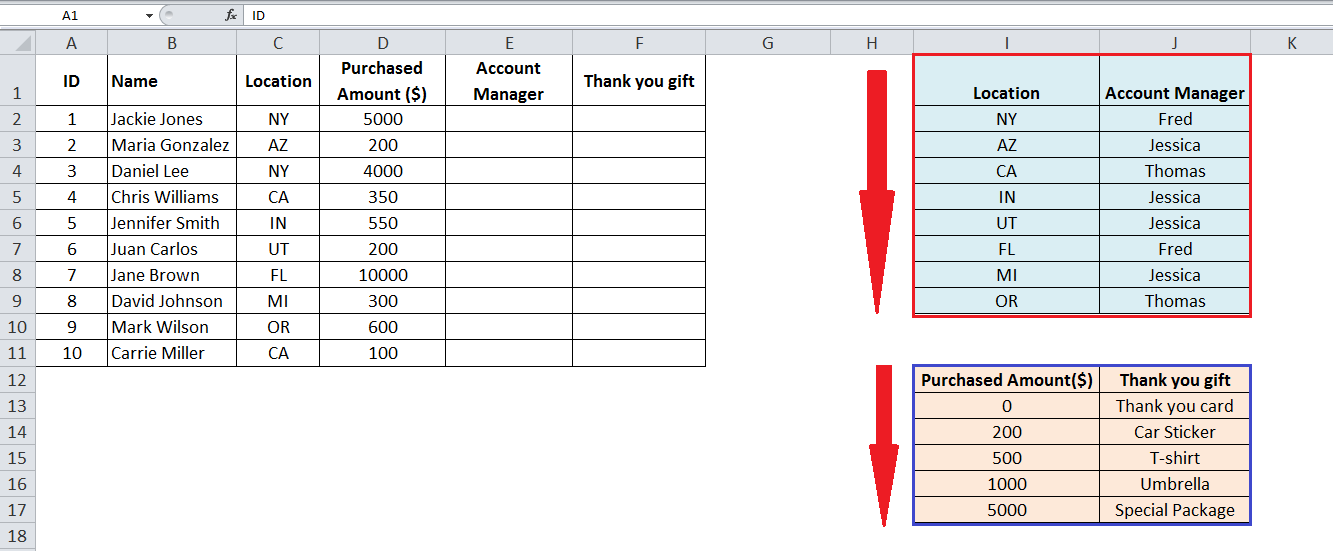You can see we have two array tables to search the values of location (I1:J9) and purchased amounts (I12:J17). Lookup values in both array tables are organized in vertical rows and we need to retrieve the information of “Account Manager” and “Thank you gift” based on location and purchased amount respectively.

### VLOOKUP for Exact match

You can look up the values based on exact match type and retrieve the information from table_array where lookup value is exactly matched. In this example, you need to do vlookup for location (lookup_value) and retrieve the Account Manager name from array table 1 (I1:J9) where location code is an exact match in the first column of array table 1.

As you need to place the missing information of Account Manager in column E, so you will insert the VLOOKUP formula in active cell E2 and will copy down the formula to other rows vertically.

`=VLOOKUP(C2,\$I\$1:\$J\$9,2,FALSE)`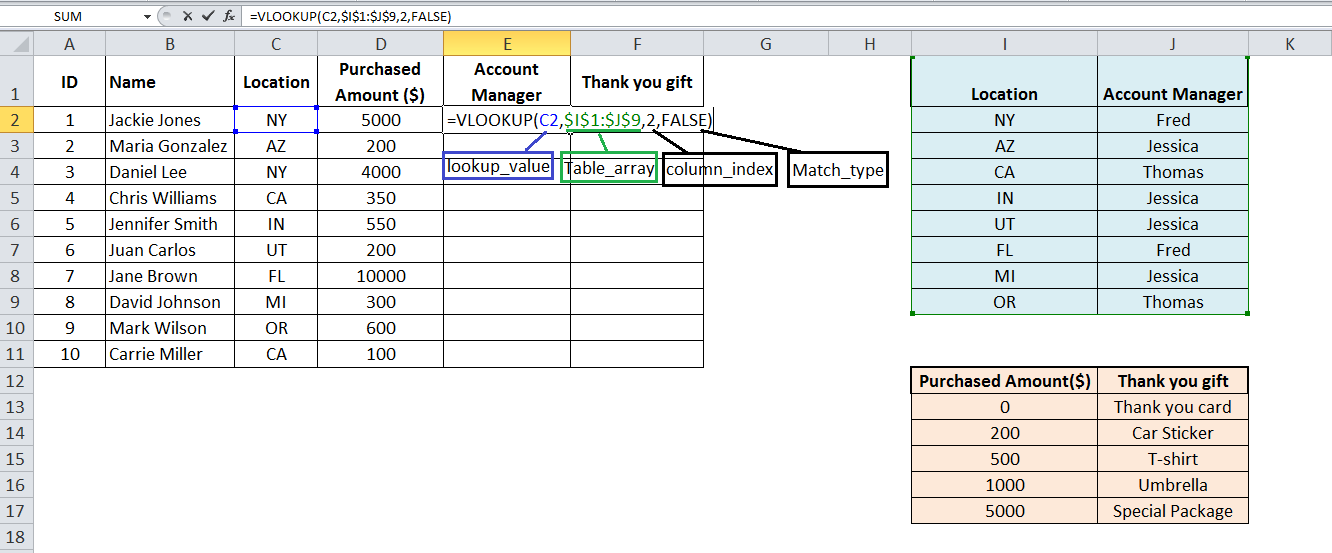As you need to search values of column C (Location) in array table 1, so C2 is the cell reference as lookup_value. You need to make the table array absolute (\$I\$1:\$J\$9) by inserting \$ sign with column and row references so that it does not change when you copy down the formula. Colum_index is 2 because “Account Manager” information is present in the 2nd column of array table 1, and range_lookup is FALSE for exact match type.

After pressing ENTER you need to copy down the formula to other rows so that all relevant information is retrieved from array table to our desired place, column E.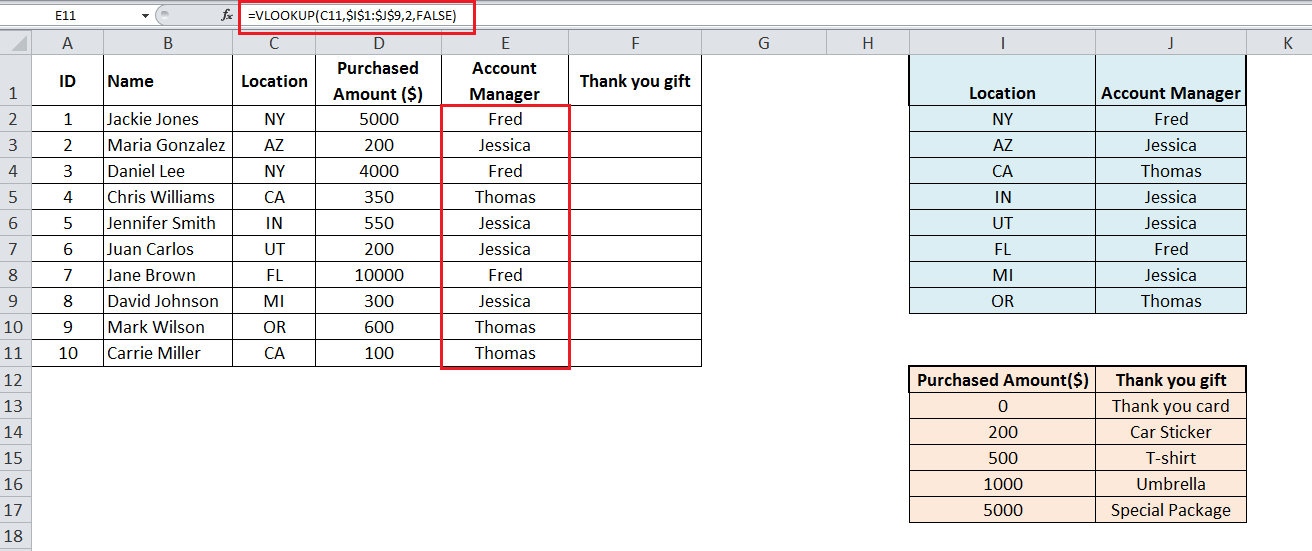### VLOOKUP for the Approximate match

You can look up the values based on approximate match type and retrieve the information from table_array where lookup value is approximately matched. In this example, you need to do vlookup for Purchased Amount (lookup_value) and retrieve the Thank You Gift item from array table 2 (I12:J17) where Purchased Amount is approximately matched in the first column of array table 2. As you need to do vlookup based on approximate match so you need to sort the array table 2 in ascending order (lowest to highest) for Purchased Amount column (first column of array table 2), like below;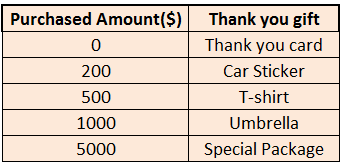As you need to place the missing information of Thank You Gift items in column F, so you will insert the VLOOKUP formula in active cell F2 and will copy down the formula to other rows vertically.

`=VLOOKUP(D2,\$I\$12:\$J\$17,2,TRUE)`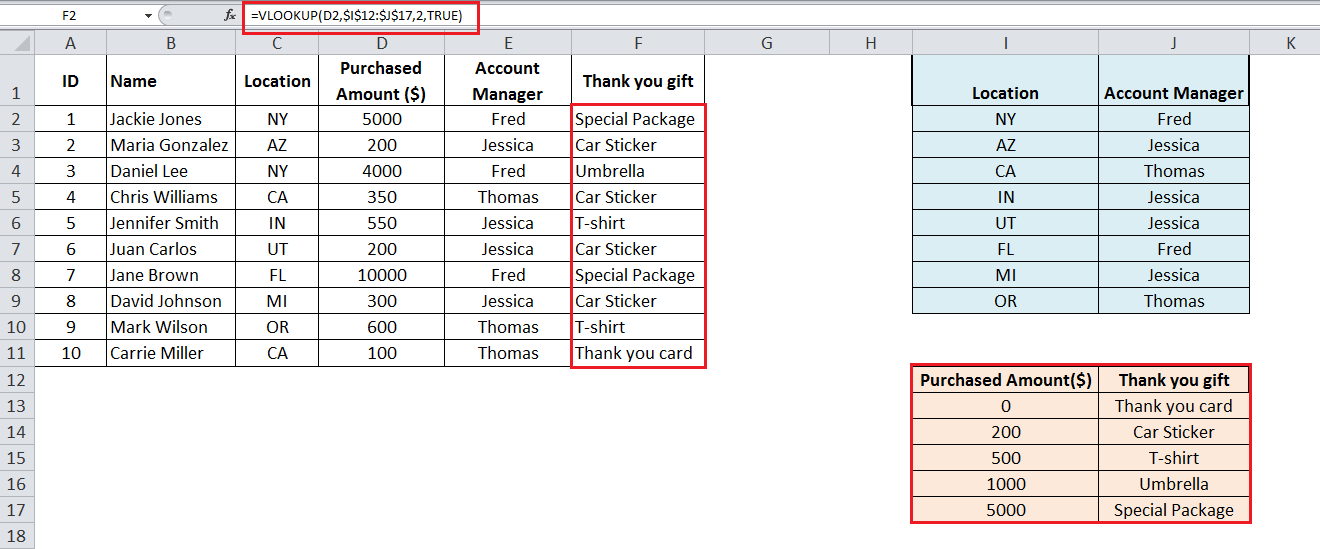As you need to search the values of Purchased Amount of column D in the first cell of array table 2 and pick the relevant gift items in column 2 of array table 2 based in an approximate match, so VLOOKUP will return next smaller value as lookup value, if there is no exact matching figure is found.

For example look at the purchased amount of \$350 in cell D5. As there is no exact matching figure available in the first column of array table 2, so Vlookup will return next smaller figure of 200 as lookup value and retrieve the gift item in next column, same row.

### Did this post not answer your question? Get a solution from connecting with the expert.

Another blog reader asked this question today on Excelchat:
Here are some problems that our users have asked and received explanations onHow do I convert text to date format?How do I convert text to date format?How do I convert text to date format?How do I convert text to date format?
Solved by X. D. in 26 minshow to do v look up, how to make amount in words,
Solved by T. J. in 27 minsHOW TO DO CHART USING EXCEL AND HOW TO CREATE A PIVOT TABLE
Solved by K. E. in 16 mins